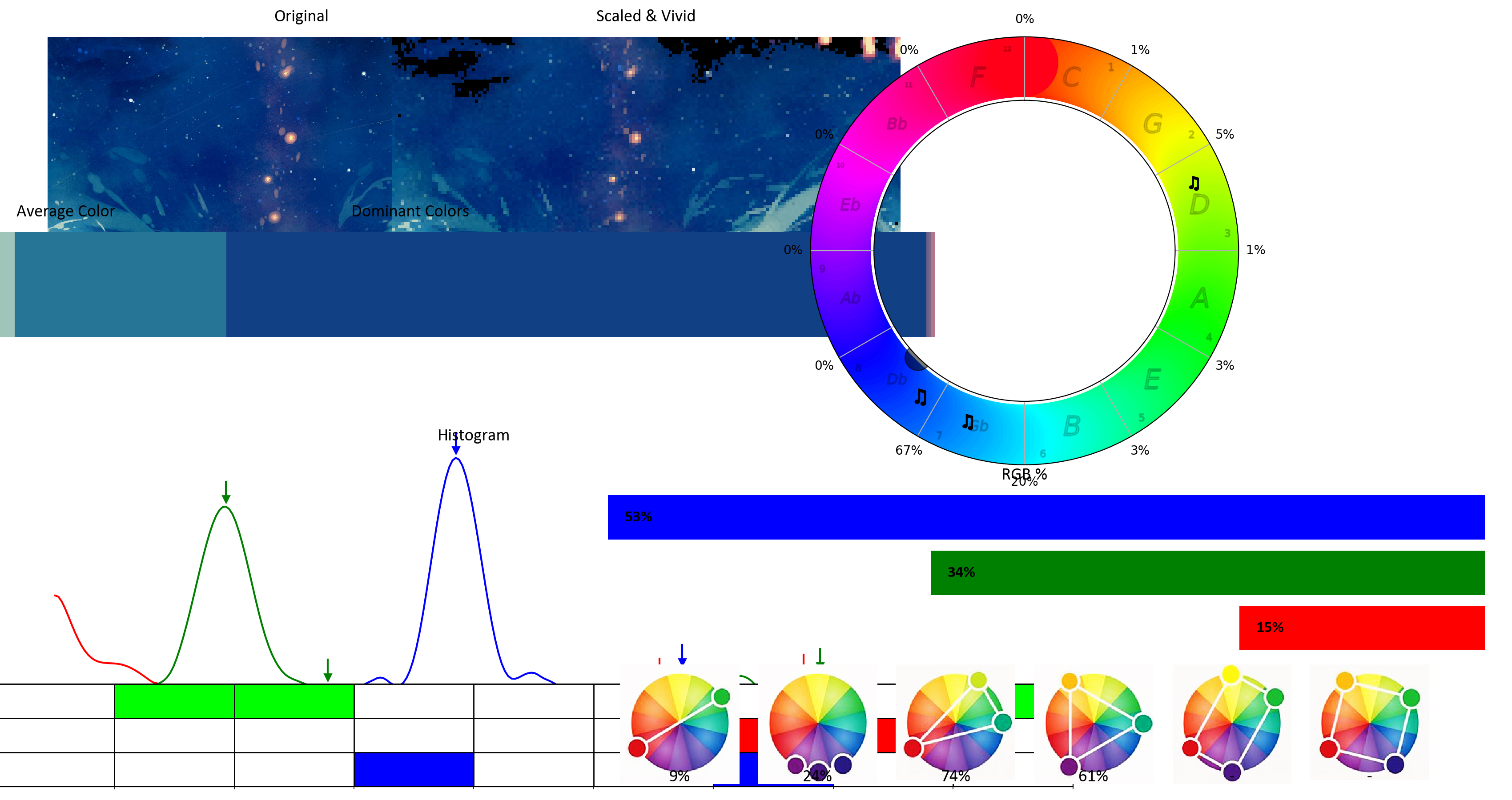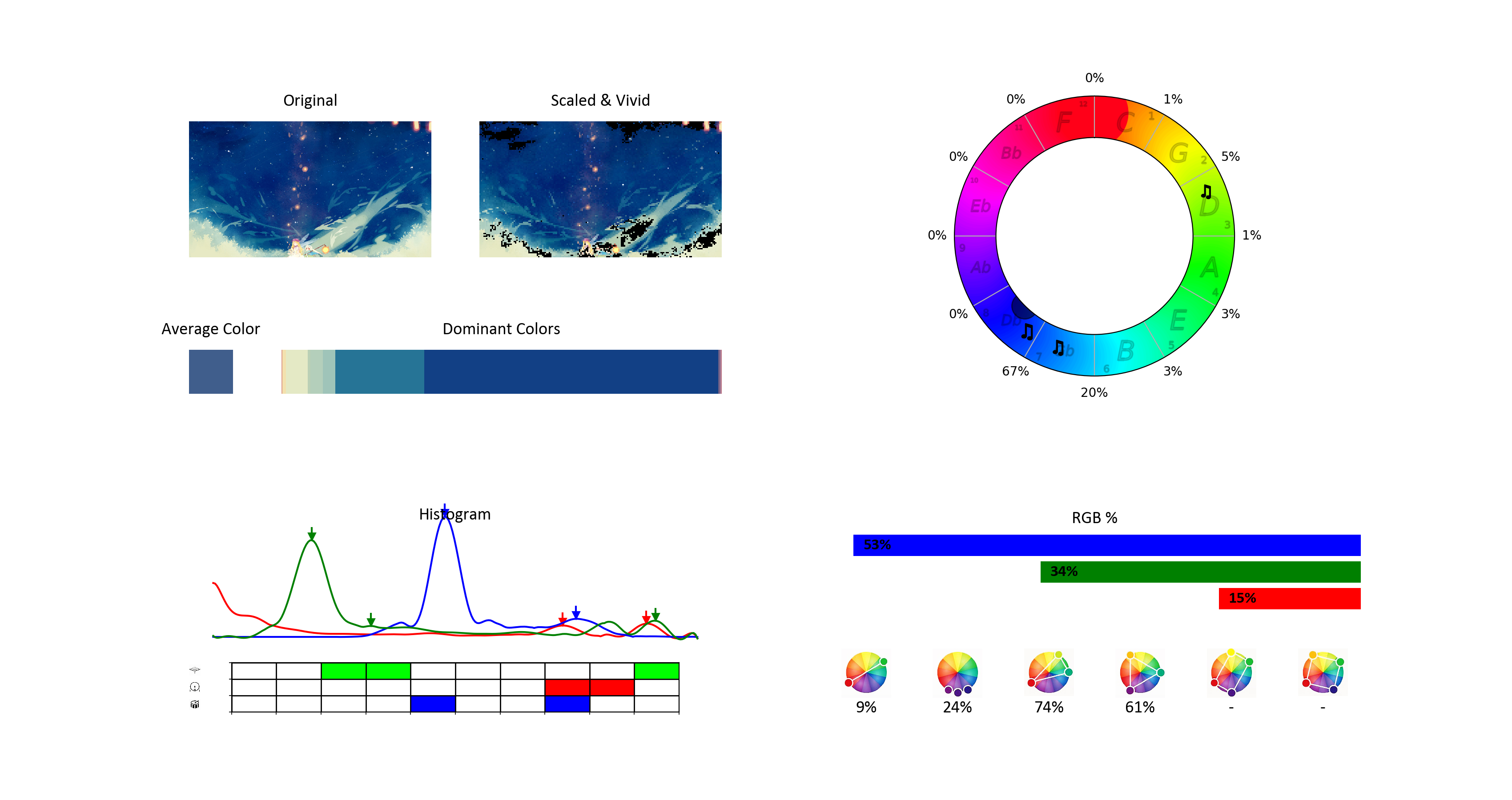I am working on a project that has some graphs that I want to place in specific positions, and I am doing so by using code configurations like

`Code:grid = gridspec.GridSpec(2, 2)inner = gridspec.GridSpecFromSubplotSpec(3, 2, subplot_spec=grid[1, 1], height_ratios=[1, 2, 1])inner2 = gridspec.GridSpecFromSubplotSpec(2, 2, subplot_spec=inner[1, 0])graph = plt.subplot(inner2)`

I had reached a point that everything look good and neat, then I did some code changes, and reached a point that all the graphs was meshed up and overlapping each other. I double checked the code and found no flaws, and then I tried to run the same code not in SciView but on the default matplotlib window. And all the graphs was correctly positioned!

### Sciview:### Matplotlib window:I think that in the mean while the PyCharm program updated from PY-183.4284.139 to PY-183.4588.64

I figured out by testing, that the sciView applies fig.tight_layout() without me having that command on the code. I think there is where problem originate, because when I add plt.tight_layout() on the code the matplotlib windows shows the graphs as the same mesh overlapping each other as well. So is there any control on whether the SciView applies tight_layout() or not?

3 commentsMay I ask you to attach a sample code (can be uploaded to https://uploads.services.jetbrains.com/ zipped) so I can do some testing on my side? I can guarantee its privacy. Just let me know the name of that zip file.Hi,

I figured out what was happening. And it was a wrong practice from my side. Still it is weird because with the same code I had different plots on SciView and the matplotlib default windows, but now I know why.  The problem was that I was using different gridspec.GridSpec , actually I was using a new gridspec.GridSpec for each graph, so at the end the program was confused of which gridspec.GridSpec to use (even if they where splitting the image to same pieces). And the tight_layout had as an outcome a mesh. I will add a sample code here with the practice that I was using (that was causing the problem) and the correct one (that does not cause the problem)

Wrong one:

`def plot_image(image):    grid = gridspec.GridSpec(1, 2)  #<----    img = plt.subplot(grid[0,0])    img.imshow(image)def plot_rgb_percents(rgb_percents):    grid = gridspec.GridSpec(1, 2)  #<----    rgb_bars = plt.subplot(grid[0,1])    r, g, b = plt.barh(['R','G','B'],[rgb_percents['r'],rgb_percents['g'],rgb_percents['b']])plt.figure(1, figsize=(100,100), dpi=100)plot_image(someImage)plot_rgb_percents(someData)plt.show()`

Correct one:

`grid = gridspec.GridSpec(1, 2) #<----def plot_image(image):    img = plt.subplot(grid[0,0])    img.imshow(image)def plot_rgb_percents(rgb_percents):    rgb_bars = plt.subplot(grid[0,1])    r, g, b = plt.barh(['R','G','B'],[rgb_percents['r'],rgb_percents['g'],rgb_percents['b']])plt.figure(1, figsize=(100,100), dpi=100)plot_image(someImage)plot_rgb_percents(someData)plt.show()`

So now you have to figure out, why in SciView when using the "wrong one" code,  it auto-applied the tight_layout() without the command being there, and why on the matplotlib default window it didn't?Thanks for letting us know!

>So now you have to figure out, why in SciView when using the "wrong one" code,  it auto-applied the tight_layout() without the command being there, and why on the matplotlib default window it didn't?

This is a known issue, unfortunately, https://youtrack.jetbrains.com/issue/PY-32223. Duplicated by https://youtrack.jetbrains.com/issue/PY-32106

Please sign in to leave a comment.